# Reflection of Light

Welcome to class!

In today’s class, we will be talking about the reflection of light. Enjoy the class!

### Reflection of LightLight as a wave exhibit wave property of reflection. When light falls on a polished surface, it is reflected in a direction depending on the angle at which it strikes the surface. There are two types of reflection, regular and diffuse. Regular reflection takes place when light falls on a smooth surface. In diffuse reflection, the rays are scattered after reflection. If the incident rays were parallel, the reflected rays will no longer be parallel. Diffuse reflection occurs when light falls on a rough or uneven surface.#### Laws of Reflection

The two simple laws governing the reflection of light are as follows:

1. The incident ray, the reflected ray and the normal, at the point of incidence, all lie in the same plane
2. The angle of incidence is equal to the angle of reflection
##### Image in a plane mirror

When an object is placed in front of a mirror, rays of light from the object incident on the mirror. The rays are then reflected to appear as if they were coming from somewhere behind the mirror-image of the object is formed at the point where the reflected ray meet or appear to meet (behind the mirror).

The image formed in a plane mirror has the following characteristics:

1. The image has the same size as the object
2. Image is laterally inverted (that is the image of letter L, for instance, appear as ┘)
3. It is virtual (cannot be focused on screen)
4. Image distance behind the mirror is equal to object distance in front of the mirror.
##### Inclined mirror

When two plane mirrors are inclined at some angles to each other, a number of images are formed. This depends on the angle of inclination according to the formula N =  (it  gives an odd number)WORKED EXAMPLE

(1) Calculate the number of images formed by two mirrors inclined at an angle 90° to each other. The object unit distance from each mirror.

SOLUTION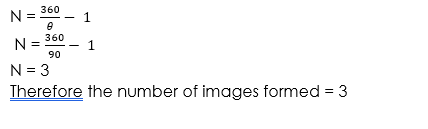(2) Suppose two plane mirrors are inclined at 60° to another, then the number of images formed is given by?

SOLUTION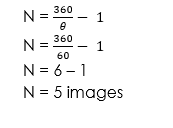Rotation of a Plane Mirror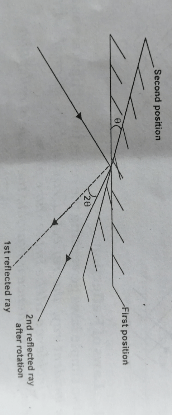When a mirror is rotated through an angle, keeping the incident ray in its position, the reflected ray will be rotated through an angle 2.

##### Reflection at curved mirrors

There are two types of curved mirrors. These are concave and convex mirrors.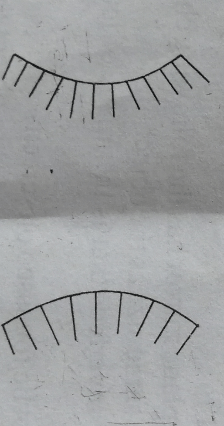Because these curved mirrors are part of a sphere we call them a spherical mirror.If you allow parallel rays of light to fall on a concave mirror, the rays will converge at one point after reflection. That point is called the focus of the mirror. Because of this property of concave mirror, it is sometimes called converging mirror.

Note the following terms in describing image formation in a concave mirror.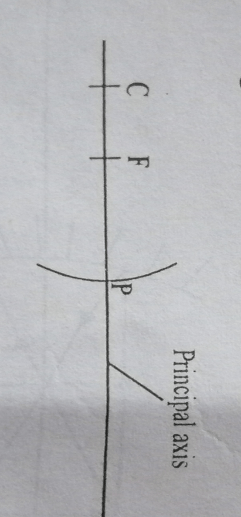1. The principal axis is the straight horizontal imaginary line passing through the pole P and the centre of curvature of the mirror.
2. Pole (P): The centre or midpoint of the reflecting surface.
3. Centre of curvature (C): The concave mirror is part of a sphere. That is, it is a surface cutout off a sphere. The centre of the sphere of which the mirror is a part is called the centre of curvature.
4. Radius of curvature: Is the distance from the mirror surface to the centre of curvature. That is the Radius of the sphere of which the mirror is a part.
5. Principal focus: The point at which parallel rays incident on the mirror meets after reflection.
6. Focal Length (f): Is the distance from the pole to the principal focus.

NOTE:

By reversibility of light, just as parallel rays incident on the concave mirror will converge at the principal focus after reflection, rays from the principal focus will be reflected parallel as shown in the diagram above.

##### Location of image for a different object position

In a concave mirror, the nature and position of the image formed depend on the position (or distance) of the object in front of the mirror.

• ###### Object at a distance greater than F###### Characteristics of the image formed
1. Inverted
2. Magnified
3. Real
4. Formed at a distance beyond ‘c’
• ###### Object at ‘c’###### Characteristics of the image formed
1. Same size as the object
2. Formed at ‘c’
3. Real
4. Inverted
• ###### Object at a distance beyond ‘c’###### Characteristics of the image formed
1. Inverted
2. Formed between ‘c’ and ‘f’
3. Real
4. Diminished
• ###### Object at ‘f’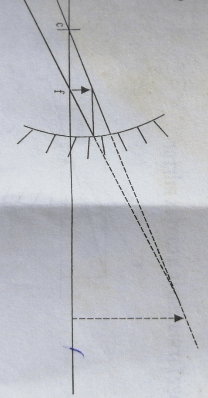###### Characteristics of the image formed
1. Erect
2. Virtual
3. Magnified
4. Formed behind the mirror
###### Uses of Concave Mirror
1. Used as a shaving mirror
2. Used as a dentist mirror.
3. Used as a reflector in car headlamp, searchlight etc. the concave mirror is slightly modified to become parabolic in shape.
##### Convex Mirror

Unlike the concave mirror in which the reflection surface curves inwards, the reflection surface of the convex mirror curve outwards. When parallel ray falls on the mirror they diverge and appear as if they are coming from a point, F behind the mirror.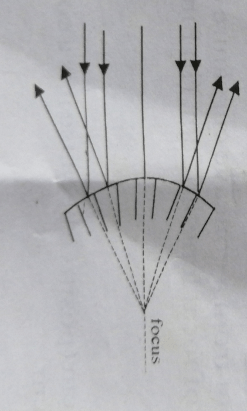Image formation in a convex mirrorNo matter the position of the object, the image formed in a convex mirror is always

1. Erect
2. Virtual
3. Diminished
###### Uses of Convex Mirror

Since the convex mirror has a wide field of view and produces an erect image at all times, it is used as a rearview mirror in cars.

###### Relationship between focal length (f) and object distance (u) and image distance (v)

That is the radius of curvature (r) is twice the focal length. In a concave or convex mirror, u, v and f are related by the equation.

1/f = 1/u + 1/v

Note:

For convex mirror f and v are virtual. Hence they are assigned a negative sign. When a concave mirror produces a virtual image v, is –ve.

WORKED EXAMPLES

(1) An object is 20 cm from a concave curvature of the mirror.

(i) What is the radius of the focal length 15cm?

(ii) Find the position and nature of the image formed.

solutionImage is 60cm in front of the mirror

(i) Image is real since v is positive

(ii) Image is formed beyond C

Magnification =  =

Image is three times bigger than the object.

(2) A dentist examining a patient’s gum, place a concave mirror of focal length 10cm close to the gum, at a distance of 8cm. determine, the position and nature of the image formed.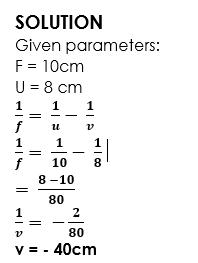(Negative sign show a virtual image, it is formed behind the mirror)

(i) Image is formed 40cm behind the mirror

(ii) It is virtual

Image is magnified

(3) A motorcycle is 10 cm behind a car from the position of the rear-view mirror. The rear-view mirror of the car has a focal length of 50cm. how far behind the car will the motorcycle appear when the driver observes from the mirror?

SOLUTION

Given parameters:

U = 10cm, f = – 50cm (rear-view of a car is convex, hence focal length is negative)In our next class, we will be talking about the Refraction of Light.  We hope you enjoyed the class.

Should you have any further question, feel free to ask in the comment section below and trust us to respond as soon as possible.

How Can We Make ClassNotesNG Better - CLICK to Tell Us💃